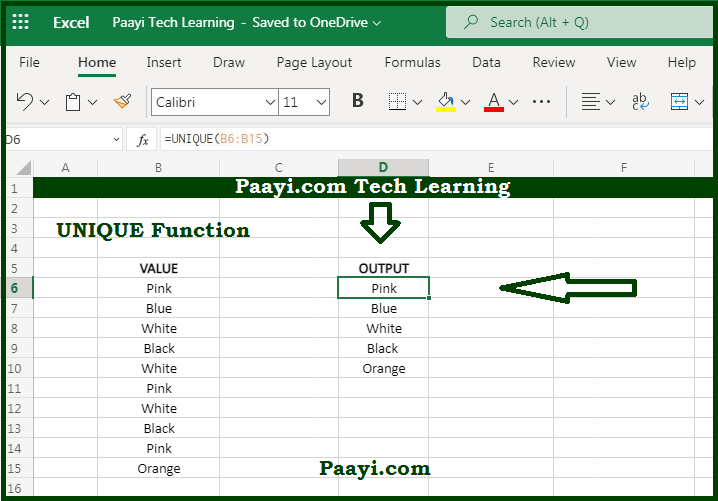# Learn How to Use Microsoft Excel UNIQUE Function

Written by | 0 Comments | 667 Views

In this article, you will learn how to use the Microsoft Excel UNIQUE function and its prime function in Microsoft Excel. You will also get to know the Microsoft Excel UNIQUE function return value and syntax with the help of some examples.

Microsoft Excel UNIQUE Function

The primary function of the Microsoft Excel UNIQUE function is to extract unique values from the range. That implies with the UNIQUE function's help, you can able to return the unique values in the list or range. The values can be numbers, texts, dates, or times, etc.

Return Value of UNIQUE Function

The return value will be the array of unique values.

Syntax of UNIQUE Function

=UNIQUE(array, [by-col], [exactly-once])

Where the arguments:

array: This is the range or array from which you want to extract the unique values.

by-col: This determine how to compare and extract, by row = FALSE (default), by column = TRUE (optional).

exacly-once: TRUE = values that occur once, FALSE = all unique values, default is FALSE (optional).

## How to Use Microsoft Excel UNIQUE Function?Now we know that the Microsoft Excel UNIQUE function is used to extract a list of unique values from a range or array. The result will be a dynamic array with a unique value. In case this array is the final result, that means not handed off to another function, the array will spill and automatically update if the new unique values are added or removed from the source range.